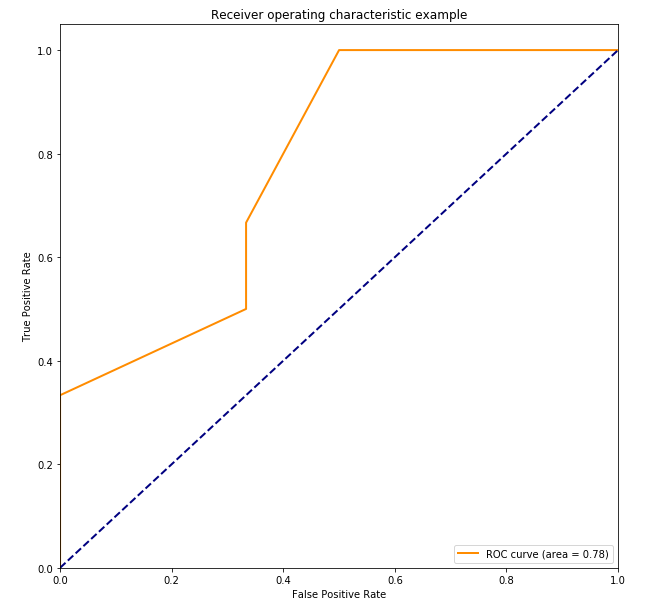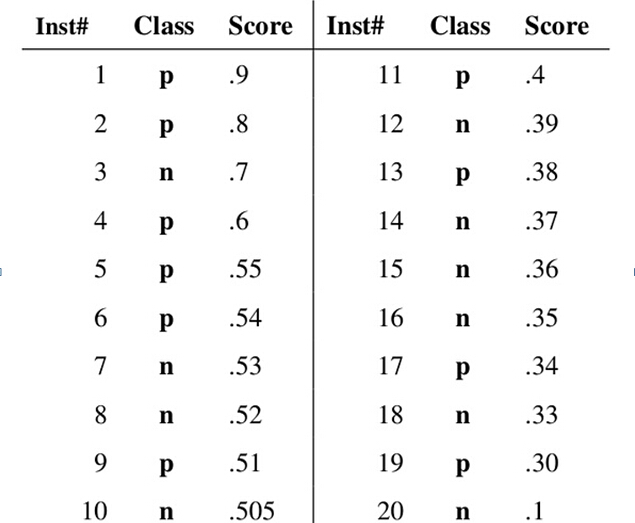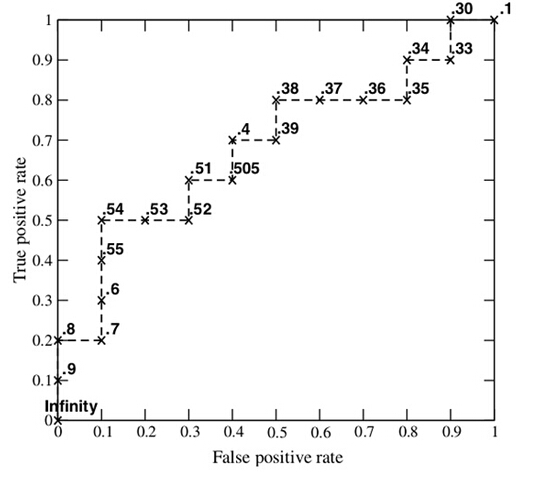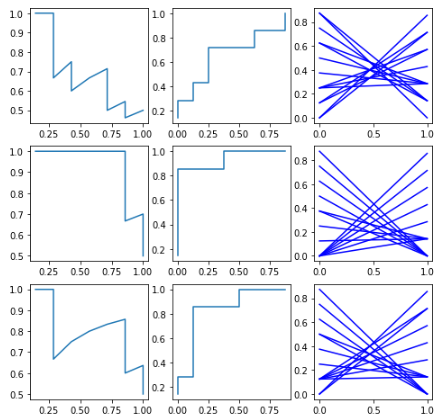• 使用Python画出ROC曲线后，如何在ROC曲线代码中增加95%CI？ 计算AUC之后，大部文献都会给出95%CI，如何在代码中增加这一功能呢？希望有大神给出代码！！！！ 代码如下： import matplotlib.pyplot as plt from ...
使用Python画出ROC曲线后，如何在ROC曲线代码中增加95%CI？
计算AUC之后，大部文献都会给出95%CI，如何在代码中增加这一功能呢？希望有大神给出代码！！！！
代码如下：
import matplotlib.pyplot as plt
from sklearn import svm
from sklearn.metrics import roc_curve, auc
import numpy as np
from sklearn import metrics
y = np.array([1,1,1,1,1,1, 2,2,2,2,2,2])
pred = np.array([0.1,0.2,0.2,0.3,0.4, 0.4, 0.3,0.5,0.4,0.3,0.35, 0.8])
fpr, tpr, thresholds = metrics.roc_curve(y, pred, pos_label=2)
metrics.auc(fpr, tpr)
plt.figure()
lw = 2
plt.figure(figsize=(10,10))
plt.plot(fpr, tpr, color=‘darkorange’,
lw=lw, label=‘ROC curve (area = %0.2f)’ % roc_auc) ###假正率为横坐标，真正率为纵坐标做曲线
plt.plot([0, 1], [0, 1], color=‘navy’, lw=lw, linestyle=’–’)
plt.xlim([0.0, 1.0])
plt.ylim([0.0, 1.05])
plt.xlabel(‘False Positive Rate’)
plt.ylabel(‘True Positive Rate’)
plt.legend(loc=“lower right”)
plt.show()展开全文python
• ROC曲线（Receiver Operating Characteeristic Curve）是显示Classification模型真正率和假正率之间折中的一种图形化方法。  解读ROC图的一些概念定义:：  真正（True Positive , TP）被模型预测为正的正样本  假...
一、基本概念

解读ROC图的一些概念定义:：
真正（True Positive , TP）被模型预测为正的正样本
假负（False Negative , FN）被模型预测为负的正样本
假正（False Positive , FP）被模型预测为正的负样本
真负（True Negative , TN）被模型预测为负的负样本 真正率（True Positive Rate , TPR）或灵敏度（sensitivity） TPR = TP /（TP + FN） 正样本预测结果数 / 正样本实际数

假负率（False Negative Rate , FNR） FNR = FN /（TP + FN）
被预测为负的正样本结果数 / 正样本实际数

假正率（False Positive Rate , FPR） FPR = FP /（FP + TN） 被预测为正的负样本结果数 /负样本实际数

真负率（True Negative Rate , TNR）或特指度（specificity） TNR = TN /（TN + FP）
负样本预测结果数 / 负样本实际数
目标属性的被选中的那个期望值称作是“正”（positive） ROC曲线上几个关键点的解释： ( TPR=0,FPR=0 ) 把每个实例都预测为负类的模型 ( TPR=1,FPR=1 ) 把每个实例都预测为正类的模型 ( TPR=1,FPR=0 ) 理想模型

一个好的分类模型应该尽可能靠近图形的左上角，而一个随机猜测模型应位于连接点（TPR=0,FPR=0）和（TPR=1,FPR=1）的主对角线上。 ROC曲线下方的面积（AUC）提供了评价模型平均性能的另一种方法。如果模型是完美的，那么它的AUG = 1，如果模型是个简单的随机猜测模型，那么它的AUG = 0.5，如果一个模型好于另一个，则它的曲线下方面积相对较大。

二、 如何画roc曲线

假设采用逻辑回归分类器，其给出针对每个实例为正类的概率，那么通过设定一个阈值如0.6，概率大于等于0.6的为正类，小于0.6的为负类。对应的就可以算出一组(FPR,TPR),在平面中得到对应坐标点。随着阈值的逐渐减小，越来越多的实例被划分为正类，但是这些正类中同样也掺杂着真正的负实例，即TPR和FPR会同时增大。阈值最大时，对应坐标点为(0,0),阈值最小时，对应坐标点(1,1)。

假设已经得出一系列样本被划分为正类的概率，然后按照大小排序，下图是一个示例，图中共有20个测试样本，“Class”一栏表示每个测试样本真正的标签（p表示正样本，n表示负样本），“Score”表示每个测试样本属于正样本的概率。接下来，我们从高到低，依次将“Score”值作为阈值threshold，当测试样本属于正样本的概率大于或等于这个threshold时，我们认为它为正样本，否则为负样本。举例来说，对于图中的第4个样本，其“Score”值为0.6，那么样本1，2，3，4都被认为是正样本，因为它们的“Score”值都大于等于0.6，而其他样本则都认为是负样本。每次选取一个不同的threshold，我们就可以得到一组FPR和TPR，即ROC曲线上的一点。这样一来，我们一共得到了20组FPR和TPR的值，将它们画在ROC曲线的结果如下图：AUC(Area under Curve)：Roc曲线下的面积，介于0.1和1之间。Auc作为数值可以直观的评价分类器的好坏，值越大越好。

首先AUC值是一个概率值，当你随机挑选一个正样本以及负样本，当前的分类算法根据计算得到的Score值将这个正样本排在负样本前面的概率就是AUC值，AUC值越大，当前分类算法越有可能将正样本排在负样本前面，从而能够更好地分类。

三、 代码实现

predict = [0.9,0.8,0.7,0.6,0.55,0.54,0.53,0.52,0.51,0.505,0.4,0.39,0.38,0.37,0.36,0.35,0.34,0.33,0.3,0.1];
ground_truth = [1,1,0,1,1,1,0,0,1,0,1,0,1,0,0,0,1,0,1,0];
auc = plot_roc(predict,ground_truth)

function auc = plot_roc(predict,ground_truth)
pos_num = sum(ground_truth==1);
neg_num = sum(ground_truth==0);

m = size(ground_truth,2);
[pre,Index] = sort(predict);
ground_truth = ground_truth(Index);
x = zeros(m+1,1);
y = zeros(m+1,1);
auc = 0;
x(1) = 1; y(1) = 1; %当阈值为0.1时，都被分类为了正类，所以真阳率和假阳率都为1.
for i = 2:m
TP = sum(ground_truth(i:m) == 1); %大于x(i)的标签为1的都是真阳，大于x(i)的标签为0的都是假阳
FP = sum(ground_truth(i:m) == 0);
x(i) = FP/neg_num;
y(i) = TP/pos_num;
auc = auc + (y(i)+y(i-1))*(x(i-1)-x(i))/2;
end

x(m+1) = 0; y(m+1) = 0;
auc = auc + y(m)*x(m)/2;%这句多余
plot(x,y);
end

predict ——分类器对于测试集的分类结果

ground_truth——测试集的正确标签，即为0和1

auc——范湖ROC曲线下的面积

转自：http://www.360doc.com/content/14/1208/23/19252401_431420662.shtml

https://blog.csdn.net/chl033/article/details/4507544

https://www.cnblogs.com/dlml/p/4403482.html
展开全文• def ROC(scores): # scores = [["p", 0.9], ["n", 0.8] ...] scores = sorted(scores, key = lambda x: -x) p = 0 n = 0 for score in scores: if score == "p": p += 1 else: n += 1 res = [] fo
图和例子可见 https://blog.csdn.net/qq_45603919/article/details/104731164
绘画ROC最直观的就是先按照概率进行排序，然后，遇到正样本就往y轴正方向前进一个单位，遇到负样本就往x轴正方向前进一个单位。当然横坐标就是负样本的个数，纵坐标就是正样本的个数。
def ROC(scores):
# scores = [["p", 0.9], ["n", 0.8] ...]
scores = sorted(scores, key = lambda x: -x)
p = 0
n = 0
for score in scores:
if score == "p":
p += 1
else:
n += 1
res = []
for i in range(len(scores)):
if i == 0:
if scores[i] == "p":
res.append([1, 0])
else:
res.append([0, 1])
else:
print(scores)
if scores[i] == "p":
a, b = res[-1]
res.append([a+1, b])
else:
a, b = res[-1]
res.append([a, b+1])
out = [[0, 0]]
for i in range(len(res)):
a, b = res[i]
out.append([a/p, b/n])
return out

scores = [["p", 0.9], ["p", 0.78], ["n", 0.6], ["p", 0.46], ["n", 0.4], ["n", 0.37], ["p", 0.2], ["p", 0.16]]
print(ROC(scores))



def PR(scores): # precision = 分对的正样本 / 真实的正样本(p); Recall； 分类正确的正样本 / 判定为正样本
scores = sorted(scores, key=lambda x: -x)
p = 0
n = 0
for score in scores:
if score == "p":
p += 1
else:
n += 1
real_p = 0
res = [[0, 1]]
for i in range(len(scores)):
if scores[i] == "p":
real_p += 1
res.append([real_p, i + 1]) # i是判定为正样本
else:
res.append([real_p, i + 1])

out = [[0, 0]]  # 注意，大于第一个case的阈值的时候，recall是0，precision为1(0/0)
for i in range(len(res)):
real_p, res_p = res[i]
out.append([real_p/p, real_p/res_p])
return out

scores = [["p", 0.9], ["p", 0.78], ["n", 0.6], ["p", 0.46], ["n", 0.4], ["n", 0.37], ["p", 0.2], ["p", 0.16]]
print(PR(scores))




展开全文• main.m”用于绘制接收器操作特性曲线（ROC曲线）和计算ROC曲线下面积（AUROC） 数字 图1不同筛选方法的ROC曲线和AUROC值我是谁 元重天博士候选医学物理实验室。 -SUMP实验室。 成均馆大学三星健康科学与技术高级研究...
• 举例说明 P-R图的计算 ROC曲线的计算 代价曲线的计算与绘制 以上三者都要求逐个把样本作为正例进行预测，模拟的是threshold设为不同值时同一个模型的预测效果 原理内容请参考《机器学习》周志华 2.3节。也可查看...
举例说明  P-R图的计算          ROC曲线的计算          代价曲线的计算与绘制
以上三者都要求逐个把样本作为正例进行预测，模拟的是threshold设为不同值时同一个模型的预测效果
原理内容请参考《机器学习》周志华  2.3节。也可查看以下网址

PR曲线和F1、ROC曲线和AUC

模型评估与选择（后篇）-代价曲线
只需要在下面的模块中更改model_sequence里面的分类序列的顺序。以下为针对某一二分类问题的三个模型的P-R图、ROC曲线、代价曲线。

P-R图，如果模型A的P-R 图将模型B的P-R图完全包住，则A效果比B好。同时还要看BEP和F1值也可以用来比较模型效果。

ROC曲线，曲线下方面积为AUC，AUC越大，模型效果越好。曲线上方部分面积为loss，loss越小模型越好。模型A的ROC曲线如果完全把模型B的ROC曲线包在下方则模型A的效果比B好。如果两者并不是完全包含与被包含的关系，而是有重叠区域也有未重叠区域，则用AUC值比较。

代价曲线是在出现非平衡代价的情况下才使用的。所有曲线最下方的面积越小，则代表模型越好。

如下9幅图为3个模型对同一个样本的分类结果。第一行为model_1的P-R图、ROC曲线、代价曲线。第二行为model_2,第三行为model_3。三个model对比下来model_2效果最好。绘制过程见下文代码。# 假设我们的样本是7个1和8个0.    1作为正例，0作为负例
model_1 = [1,1,0,1,0,1,1,0,0,0,1,0,0,1,0]
model_2 = [1,1,1,1,1,1,0,0,0,1,0,0,0,0,0]
model_3 = [1,1,0,1,1,1,1,0,0,0,1,0,0,0,0]
model_sequence = [model_1,model_2,model_3]

def PNTF(model_sequence, n):# 将第几个样本之前的全部作为正例。n是从1开始的，不是从0开始的
count = len(model_sequence)
class_1_count = sum(model_sequence)
class_0_count = count - class_1_count
if (n>count - 1) or (n<1):
return None
else:
TP = sum(model_sequence[0:n])
FN = class_1_count - TP
FP = n - TP
TN = class_0_count - FP
return TP,FN,TN,FP

def P_R(TP,FN,TN,FP):
P = TP/(TP+FP)
R = TP/(TP+FN)
F1 = 2*P*R/(P+R)
return P,R,F1

def ROC_TPR_FPR(TP,FN,TN,FP):
TPR = TP/(TP+FN)
FPR = FP/(TN+FP)
return TPR,FPR

def cost_curve_FPR_FNR(TP,FN,TN,FP):
TPR = TP/(TP+FN)
FNR = 1- TPR#假反率
FPR = FP/(TN+FP)
return FPR,FNR

#只是用于test
def PR_ROC_Cost_Curve_public_part(model_sequence,verbose = False):
length = len(model_sequence)
if verbose==True:
print('把前n个样本作为正例进行预测')
print('n\t','TP\t','FN\t','TN\t','FP\t','P\t','R\t','F1\t','TPR\t','FPR\t','FNR')
list_P_R_F1 = []
list_ROC_TPR_FPR = []
list_cost_curve_FPR_FNR = []
for i in range(length - 1):
TP,FN,TN,FP = PNTF(model_sequence,i+1)
P,R,F1 = P_R(TP,FN,TN,FP)
TPR,FPR = ROC_TPR_FPR(TP,FN,TN,FP)
FPR,FNR = cost_curve_FPR_FNR(TP,FN,TN,FP)
if verbose ==True:
print(i+1,'\t',TP,'\t',FN,'\t',TN,'\t',FP,'\t',round(P,3),'\t',round(R,3),'\t',round(F1,3),'\t',round(TPR,3),'\t',FPR,'\t',round(FNR,3))
list_P_R_F1.append([P,R,F1])
list_ROC_TPR_FPR.append([TPR,FPR])
list_cost_curve_FPR_FNR.append([FPR,FNR])
return list_P_R_F1,list_ROC_TPR_FPR,list_cost_curve_FPR_FNR

import matplotlib.pyplot as plt
import numpy as np
model_count = len(model_sequence)
fig,axis = plt.subplots(model_count,3,figsize = (8,8))
axis = axis.ravel()
count = 0
for i in range(model_count):
list_P_R_F1,list_ROC_TPR_FPR,list_cost_curve_FPR_FNR = PR_ROC_Cost_Curve_public_part(model_sequence[i])
array_P_R_F1 = np.array(list_P_R_F1)
P = array_P_R_F1[:,0]
R = array_P_R_F1[:,1]
axis[count].plot(R,P)
count +=1

array_ROC_TPR_FPR = np.array(list_ROC_TPR_FPR)
TPR = array_ROC_TPR_FPR[:,0]
FPR = array_ROC_TPR_FPR[:,1]
axis[count].plot(FPR,TPR)
count +=1

array_cost_curve_FPR_FNR = np.array(list_cost_curve_FPR_FNR)
FPR = array_cost_curve_FPR_FNR[:,0]
FNR = array_cost_curve_FPR_FNR[:,1]
for j in range(len(FPR)):
axis[count].plot((0,1),(FPR[j],FNR[j]),'b')
count +=1


展开全文机器学习
• ROC曲线的横轴为假正例率(FPR), 纵轴为真正例率(TPR)，其曲线下面的面积为AUC FPR = FP/(FP+TN) TPR = TP/(TP+FN) 绘制ROC曲线的关键是弄懂ROC曲线的含义，然后改变分类概率的阈值。 代码说明： predict是用...python
• 登录注册写文章首页下载APP机器学习基础（1）- ROC曲线理解蘑菇轰炸机关注赞赏支持机器学习基础（1）- ROC曲线理解22018.09.09 16:14:12字数 2776阅读 8325本文用于理解ROC曲线的定义，绘制过程及其应用实现。...
• 原理 ROC 全称是"受试者工作特征" (Receiver Operating Characteristic) ...ROC 曲线"。 ROC 曲线的纵轴是"真正例率" (True Positive Rate，简称 TPR)，横轴是"假正例率" (Fa...
• ## 如何画ROC曲线和FROC曲线

万次阅读 热门讨论 2019-04-07 20:06:26
ROC曲线代码 具体去看https://www.jianshu.com/p/5df19746daf9。，里面的代码讲的详细 例子 # coding=UTF-8 from sklearn import metrics import matplotlib.pylab as plt #真实值 GTlist = [1.0, 1.0, 0.0, ...
• roc曲线和auc ROC曲线 (The ROC Curve) The receiver operating characteristic (ROC) curve is frequently used for evaluating the performance of binary classification algorithms. It provides a graphical ...python
• 文章目录Roc曲线和PR曲线的理解及简单的代码实现1.引言2.几个度量的介绍与理解3.PR曲线的理解4.Roc曲线的理解5.简要代码绘制两种曲线 Roc曲线和PR曲线的理解及简单的代码实现 1.引言 Roc曲线和PR曲线常被用来在二...机器学习
• ## sklearn ROC曲线使用

万次阅读 多人点赞 2019-03-03 09:10:13
目录ROC曲线定义绘制ROC曲线AUC定义代码讲解二分类多分类 这篇文章中我将使用sklearn的ROC曲线官方示例代码进行讲解，当然主要目的还是在于记录，好记性不如烂键盘嘛。 ROC曲线定义 ROC曲线是Receiver Operating ...二分类 评价指标
• ## 多分类下的ROC曲线和AUC

万次阅读 多人点赞 2018-03-05 17:37:20
本文主要介绍一下多分类下的ROC曲线绘制和AUC计算，并以鸢尾花数据为例，简单用python进行一下说明。如果对ROC和AUC二分类下的概念不是很了解，可以先参考下这篇文章：...评价指标
• 本文用于理解ROC曲线的定义，绘制过程及其应用实现，主要用于自我温习回顾基础 基本目录如下： 什么是ROC曲线？ 1.1 ROC曲线的历史 1.2 ROC曲线的定义 1.3 ROC曲线的应用场景 如何绘制ROC曲线？ 2.1 ...
• 答案是可以利用roc曲线来确定比较好的划分阈值。 ROC曲线介绍 二分类过程，设定阈值，大于该分数为1，小于该分数为0，统计计算TP， FN， FP，TN等数据计算FPR，TPR p(positive): 标签1 n(negative): 标签0 t(true)...阈值
• 在MATLAB中绘制ROC曲线，可以对分类器的性能进行评价。
• 这篇文章将先简单的介绍ROC和AUC，而后用实例演示如何python作出ROC曲线图以及计算AUC。AUC介绍AUC(Area Under Curve)是机器学习二分类模型中非常常用的评估指标，相比于F1-Score对项目的不平衡有更大...
• 使用matlab画ROC曲线，% predict - 分类器对测试集的分类结果 % ground_truth - 测试集的正确标签,这里只考虑二分类，即0和1 % auc - 返回ROC曲线的曲线下的面积
• ROC曲线是评估模型效果的重要工具，其X轴为假阳性率，Y轴为真阳性率（也叫召回率recall），其意义在于，在真阳性率时，模型同时判错阳性的样本比例，因此曲线越陡，越表示模型效果好。ROC曲线下AUC面积越大表示模型...
• ## roc曲线简单介绍及实例代码

千次阅读 热门讨论 2018-08-16 15:29:05
今日笔记：roc曲线 还是仅供自己参考学习。。 首先要注意的是roc曲线仅适用于二分类问题，不是二分类问题应首先通过各种手段转为二分类问题。 roc横坐标为TPR，纵坐标为TPR，若要知道TPR,FPR，就要从混淆矩阵说起...
• ROC曲线(Receiver Operating Characteeristic Curve) 主要是根据混淆矩阵得出来的： 真正（True Positive , TP）被预测模型为正的正样本 假负（False Negative , FN）被模型预测为负的正样本 假正（False Positive ,...机器学习
• ROC曲线（Receiver Operating Characteristic Curve）是利用Classification模型真正率（True Positive Rate）和假正率（False Positive Rate）作为坐标轴，图形化表示分类方法的准确率的高低。 ROC图的一些概念...
• 本文首先从整体上介绍ROC曲线、AUC、Precision、Recall以及F-measure，然后介绍上述这些评价指标的有趣特性，最后给出ROC曲线的一个Python实现示例。一、ROC曲线、AUC、Precision、Recall以及F-measure二分类问题的...
• http://scikit-learn.org/stable/modules/generated/sklearn.metrics.roc_curve.html http://scikit-learn.org/stable/auto_examples/model_selection/plot_roc.html#sphx-glr-auto-examples-model-selectio...